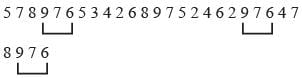Olympiad Test: Number Ranking Test - 1

# Olympiad Test: Number Ranking Test - 1

Test Description

## 10 Questions MCQ Test Mathematics Olympiad Class 7 | Olympiad Test: Number Ranking Test - 1

Olympiad Test: Number Ranking Test - 1 for Class 7 2023 is part of Mathematics Olympiad Class 7 preparation. The Olympiad Test: Number Ranking Test - 1 questions and answers have been prepared according to the Class 7 exam syllabus.The Olympiad Test: Number Ranking Test - 1 MCQs are made for Class 7 2023 Exam. Find important definitions, questions, notes, meanings, examples, exercises, MCQs and online tests for Olympiad Test: Number Ranking Test - 1 below.
 1 Crore+ students have signed up on EduRev. Have you?
Olympiad Test: Number Ranking Test - 1 - Question 1

### Direction: Study the given number series and answer the questions based on it. 5 7 8 9 7 6 5 3 4 2 6 8 9 7 5 2 4 6 2 9 7 6 4 7 8 9 7 6  Q. How many 7s are preceded by 9 and followed by 6?

Detailed Solution for Olympiad Test: Number Ranking Test - 1 - Question 1Olympiad Test: Number Ranking Test - 1 - Question 2

### Direction: Study the given number series and answer the questions based on it. 5 7 8 9 7 6 5 3 4 2 6 8 9 7 5 2 4 6 2 9 7 6 4 7 8 9 7 6  Q. Which digits have equal frequency?

Detailed Solution for Olympiad Test: Number Ranking Test - 1 - Question 2

2, 5, 8 all have frequency 3.

Olympiad Test: Number Ranking Test - 1 - Question 3

### Direction: Study the given number series and answer the questions based on it. 5 7 8 9 7 6 5 3 4 2 6 8 9 7 5 2 4 6 2 9 7 6 4 7 8 9 7 6  Q. Which digit has highest frequency?

Detailed Solution for Olympiad Test: Number Ranking Test - 1 - Question 3

7 has highest frequency.

Olympiad Test: Number Ranking Test - 1 - Question 4

Direction: Study the given number series and answer the questions based on it.
5 7 8 9 7 6 5 3 4 2 6 8 9 7 5 2 4 6 2 9 7 6 4 7 8 9 7 6
Q. Which digit has lowest frequency?

Detailed Solution for Olympiad Test: Number Ranking Test - 1 - Question 4

3 has lowest frequency.

Olympiad Test: Number Ranking Test - 1 - Question 5

Direction: Study the given number series and answer the questions based on it.
5 7 8 9 7 6 5 3 4 2 6 8 9 7 5 2 4 6 2 9 7 6 4 7 8 9 7 6
Q. Which digit occurs five times?

Detailed Solution for Olympiad Test: Number Ranking Test - 1 - Question 5

6 occurs five times.

Olympiad Test: Number Ranking Test - 1 - Question 6

Direction: Study the given number series and answer the questions based on it.
5 7 8 9 7 6 5 3 4 2 6 8 9 7 5 2 4 6 2 9 7 6 4 7 8 9 7 6
Q. How many times an even number is preceded by an odd number?

Olympiad Test: Number Ranking Test - 1 - Question 7

Direction: Study the given number series and answer the questions based on it.
5 7 8 9 7 6 5 3 4 2 6 8 9 7 5 2 4 6 2 9 7 6 4 7 8 9 7 6
Q. How many times two consecutive digits are odd numbers?

Olympiad Test: Number Ranking Test - 1 - Question 8

Direction: Study the given number series and answer the questions based on it.
5 7 8 9 7 6 5 3 4 2 6 8 9 7 5 2 4 6 2 9 7 6 4 7 8 9 7 6
Q. How many times digit 9 occurs?

Olympiad Test: Number Ranking Test - 1 - Question 9

Direction: Study the following five numbers and answer the questions given below:
617, 325, 639, 841, 592
Q. What will be the first digit of the second highest number after the positions of only the second and third digits within each number are interchanged?

Detailed Solution for Olympiad Test: Number Ranking Test - 1 - Question 9

671, 352, 693, 814, 529
The numbers in the descending order, 814, 693, 671, 529, 352
Second highest number = 693
Its 1st digit = 6

Olympiad Test: Number Ranking Test - 1 - Question 10

Direction: Study the following five numbers and answer the questions given below :
617, 325, 639, 841, 592
Q. What will be the last digit of the third number from bottom when they are arranged in descending order after reversing the position of the digits within each number?

Detailed Solution for Olympiad Test: Number Ranking Test - 1 - Question 10

716, 523, 936, 148, 295
The numbers in the descending order 936, 716, 523, 295, 148.
Third number from bottom = 52 3